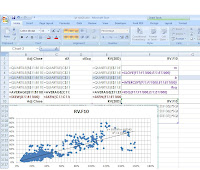Friday, November 23, 2012

Excel Data Analysis 3.2 (Volatility Forecasting off Linear Regression)

Today we look at forecasting RV( Realized Volatility) using linear regression for stock/index options trading. Generally speaking, an edge in estimating future realized volatility can be realized in units of vega off traded options; see Derman's paper, Trading Volatility, for details.

I'm still using the IYR data, and have updated the data (manually) up to Nov. 21, 2012.

Setting up linear regression

We need to match each realized volatility value from each day (row) with the future volatility, in this case I've picked the next 10 day. The calculation is exactly like the 20Day realized volatility, just instead of the past 20 days squared returns, we're using the coming 10days into the future. Logically, this column completes 10 trading days prior.

The future 10 day realized volatility is placed in the column F, RV.F10

Next we highlight the columns going from (E10, F10) down to the last row of RV.F10 at (E3127, F3127), and use the scatter plot.
Great, now the graph is up, we will have the 20Day RV on the X-axis, and RV.F10 on the Y-axis. Onto the regression fits, we right click the data on the chart and choose "add trendline". In the trendline menu, we want "Linear" type, and display "Equation on chart", "R-squared value on chart".

Remember that awesome Slope-Intercept Form, Y = mX + b, back from basic algebra? Well here it is again in the equation. As for the R-squared value, or Coefficient of Determination, the higher it is the better the equation represents the forecasting relationship.Adaptive slope/intercept  values can be utilized as we update the excel worksheet day to day. This is so we wouldn't need to plot a graph every time for these values.

And we can see that the numbers are identical.

Great, now we can create a column, G, for realized volatility forecast values, I've named it FC(RV,10). Each day's next 10Day RV forecast would use the latest day's 20Day RV with the slope + intercept values.

So let's see how much of an edge this model offers us.

We can a column in H, for the day to day change in RV, named dRV. Then in columns I, named RV.u(FC) we can check each day's change in realized vol (dRV) if the previous day's forecast was higher than the previous day's ending RV. Vice versa for column J.

Note that in the if statement, I've used "" for value if false. Basically, it either gives the dRV value, or leaves it blank if false.

We can see from the average values that both directional forecasts had a slight edge, with the downside slightly more. Therefore in actual trading, I would not rely on this edge alone, but use it as a baseline bias for a feel of coming volatility.